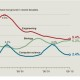## Archive for October 6, 2011

### Fixing Our Math Education with Context

Sounds pretty similar to the contextualized computing education that we’ve been arguing for with IPRE and Media Computation.  The argument being made here is another example of the tension between the cognitive (abstract conceptual learning) and the situative (integrating students into a community of practice).

A math curriculum that focused on real-life problems would still expose students to the abstract tools of mathematics, especially the manipulation of unknown quantities. But there is a world of difference between teaching “pure” math, with no context, and teaching relevant problems that will lead students to appreciate how a mathematical formula models and clarifies real-world situations. The former is how algebra courses currently proceed — introducing the mysterious variable x, which many students struggle to understand. By contrast, a contextual approach, in the style of all working scientists, would introduce formulas using abbreviations for simple quantities — for instance, Einstein’s famous equation E=mc2, where E stands for energy, m for mass and c for the speed of light.

Imagine replacing the sequence of algebra, geometry and calculus with a sequence of finance, data and basic engineering. In the finance course, students would learn the exponential function, use formulas in spreadsheets and study the budgets of people, companies and governments. In the data course, students would gather their own data sets and learn how, in fields as diverse as sports and medicine, larger samples give better estimates of averages. In the basic engineering course, students would learn the workings of engines, sound waves, TV signals and computers. Science and math were originally discovered together, and they are best learned together now.

Join 9,001 other followers

## Recent Posts

 Computing Teachers a… on Computing Teachers are Differe…Mark Guzdial on Media Computation today: Runes…Ranjidha on Media Computation today: Runes…Media Computation to… on Let’s program in social studie…Media Computation to… on Task-specific programming for…